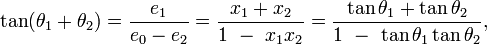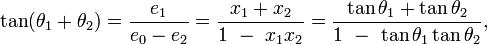# Integral Calculus

planauts

## Homework Statement

Find the values of a > 0, such that $$\int_{a }^{a^2} \frac{1}{1+x^2} dx = 0.22$$

## The Attempt at a Solution

I integrated it and got:

$$\int_{a }^{a^2} \frac{1}{1+x^2} dx = 0.22$$

$$arctan(a^2) - arctan(a) = 0.22$$

I am stuck after this, how would I solve this :-/

Thanks

Homework Helper
Just apply <tangent> on both sides of your equation, use some trigonometry and see what you get

planauts
$$a^2 - a - tan(0.22) = 0$$
I got 1.188 and -0.188
The correct answer is a = 2.04, 2.62

Homework Helper
Gold Member
You might want to look up (or prove) the correct identity for $$\tan (A-B)$$.

planauts
You might want to look up (or prove) the correct identity for $$\tan (A-B)$$.

I don't think that the tangent is the problem. I think my equation is wrong for some reason. Because when I graphed it the points don't make sense.

Do you see anything wrong with my math (integrating 1/(1+x^2) etc.)?

Homework Helper
The integration is ok. The trigonometry is the issue.

planauts
The integration is ok. The trigonometry is the issue.

Then why doesn't the answer from the key work in the equation :-/

Homework Helper
Gold Member
Then why doesn't the answer from the key work in the equation :-/

Homework Helper
Let's try it with 2.04

Equation proofing is: arctan (2.04^2) = 76.488 deg. arctan 2.04 = 63.886 deg. Difference is 13.602 degrees = 0.23 radians.

So it's correct. The other solution can be checked for accuracy in the same manner.

planauts
Let's try it with 2.04

Equation proofing is: arctan (2.04^2) = 76.488 deg. arctan 2.04 = 63.886 deg. Difference is 13.602 degrees = 0.23 radians.

So it's correct. The other solution can be checked for accuracy in the same manner.

You are saying that $$arctan(a^2) - arctan(a) = 0.22$$ is the correct equation.

So that's good. But now the problem is manipulating the equation to get the value of a.
How would I do that?

You said:
Just apply <tangent> on both sides of your equation, use some trigonometry and see what you get

I think that is where I made the mistake...I have to apply <tangent> to the whole "left hand" side as a one. I split the tangent and distributed it. I don't think that is allowed.

So if I change it: $$tan[arctan(a^2) - arctan(a)] = tan(0.22)$$

Is this what fzero meant?$$\frac{a^2 - a}{1 - a^2 * a} = \frac{a^2 - a}{1 - a^3} = 0.22$$

$$a^2 - a = 0.22 (1 - a^3)$$
$$0 = 0.22 - 0.22 a^3 - a^2 + a$$

I got a = 1 OR a = -5.3588 OR a = -0.2866

Last edited:
Homework Helper
Gold Member
Is this what fzero meant?Yes but

$$\tan(\theta_1 - \theta_2 ) = \frac{\tan \theta_1 - \tan\theta_2}{1 + \tan \theta_1 \tan\theta_2},$$

so you should find

$$\frac{a^2 - a}{1 \mathbf{+} a^2 * a} = \frac{a^2 - a}{1 \mathbf{+} a^3} = 0.22$$

Homework Helper
Looks likes a nasty cubic one gets. Cardano's formulae should be used to solve it or Maple, Mathematica if you have them.

planauts
Yes but

$$\tan(\theta_1 - \theta_2 ) = \frac{\tan \theta_1 - \tan\theta_2}{1 + \tan \theta_1 \tan\theta_2},$$

so you should find

$$\frac{a^2 - a}{1 \mathbf{+} a^2 * a} = \frac{a^2 - a}{1 \mathbf{+} a^3} = 0.22$$

I got -0.184556093 and 1.946862606 = a, which still isn't right :(

Homework Helper
Yes but

$$\tan(\theta_1 - \theta_2 ) = \frac{\tan \theta_1 - \tan\theta_2}{1 + \tan \theta_1 \tan\theta_2},$$

so you should find

$$\frac{a^2 - a}{1 \mathbf{+} a^2 * a} = \frac{a^2 - a}{1 \mathbf{+} a^3} = 0.22$$

I got -0.184556093 and 1.946862606 = a, which still isn't right :(

There's still a little mistake there. You are taking tangent value on both sides, remember? :)

So, it should read something like this:
$$\tan \left( \arctan(a ^ 2) - \arctan(a) \right) = \tan (0.22)$$
$$\Leftrightarrow \frac{a ^ 2 - a}{1 + a ^ 3} = \tan (0.22)$$.

Can you take it from here? :)

And, by the way, the book seems to have missed one possible value for a.

planauts
There's still a little mistake there. You are taking tangent value on both sides, remember? :)

So, it should read something like this:
$$\tan \left( \arctan(a ^ 2) - \arctan(a) \right) = \tan (0.22)$$
$$\Leftrightarrow \frac{a ^ 2 - a}{1 + a ^ 3} = \tan (0.22)$$.

Can you take it from here? :)

And, by the way, the book seems to have missed one possible value for a.

Oh my god! Thank you so much. I don't know why I wasn't seeing this...
I got 2.61737, 2.0416455 AND -0.223619421 as the roots.

Thanks to everyone who helped me, especially fzero :)

Homework Helper
Oh my god! Thank you so much. I don't know why I wasn't seeing this...
I got 2.61737, 2.0416455 AND -0.223619421 as the roots.

Thanks to everyone who helped me, especially fzero :)

Yup, looks good to me.

However, since the problem just asks for positive values of a (a > 0), so you don't need to take a = -0.223619421 into consideration.